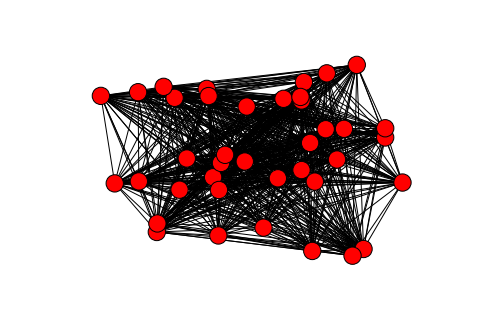# Research Question¶

What does linguistic variation between bible books tell us about their origin, and about the evolution and transmission of their texts?

# Method¶

We study the co-occurrences of lexemes across the books of the bible and represent this data in a undirected weighted graph, where the books are nodes. There are edges between very pair of books that share a lexeme occurrence. Edges are weighted: the more lexemes are shared by a pair of books, the heavier the edge. However, the weight is corrected and normalized as well.

• correction: frequent lexemes contribute less to the weight than rare lexemes,
• normalization: the weight contribution of a lexeme is divided by the number of lexemes in the union of two books.

The initial plan was to consider only common nouns, but we are also experimenting with nouns in general, verbs, and all lexemes. Moreover, we also experiment with two measures of normalisation:

• normal: divide by the sum of the number of distinct lexemes in the concatenation of two books
• quadratic: as in normal, but divide by the square of the sum.

More formally:

Let $B$ be the set of books in the Bible.

The support of a lexeme $l$ is defined as $S(l) = card\{b \in B\ \vert\ l \in b\}$.

The lexeme content of book $b$ is defined as $L(b) = \{l\ \vert\ l \in b\}$,

and the lexeme content of two books $b_1$ and $b_2$ is defined as $L(b_1, b_2) = L(b_1)\ \cup\ L(b_2)$.

The cooccurrency of those two books is defined as $C(b_1, b_2) = L(b_1)\ \cap\ L(b_2)$.

We now define two measures of weight of a cooccurrences edge between two books $b_1$ and $b_2$:

$$W_1(b_1,b_2) = {\sum \{{1\over S(l)}\ \vert\ l \in C(b_1, b_2)\} \over card\,L(b_1, b_2)}$$$$W_2(b_1,b_2) = {\sum \{{1\over S(l)}\ \vert\ l \in C(b_1, b_2)\} \over (card\,L(b_1, b_2))^2}$$

# Compute¶

Import the python modules, the plot modules, the LAF-Fabric module (laf) and initialize the laf processor.

In :
import sys
import collections
import matplotlib.pyplot as plt
from laf.fabric import LafFabric
fabric = LafFabric()

  0.00s This is LAF-Fabric 4.4.7



Load the data, especially the features we need. Note that the task will be named cooccurrences. After loading we retrieve the names by which we can access the various pieces of the LAF data.

In :
fabric.load('etcbc4', '--', 'cooccurrences', {
"xmlids": {"node": False, "edge": False},
"features": ("otype sp lex_utf8 book", ""),
})
exec(fabric.localnames.format(var='fabric'))

  0.00s LOADING API: please wait ...
0.10s INFO: USING DATA COMPILED AT: 2014-07-23T09-31-37
2.91s LOGFILE=/Users/dirk/Dropbox/laf-fabric-output/etcbc4/cooccurrences/__log__cooccurrences.txt
2.91s INFO: DATA LOADED FROM SOURCE etcbc4 AND ANNOX -- FOR TASK cooccurrences AT 2014-11-12T10-05-55


• NN: iterator of nodes in primary data order
• F: feature data

You can inspect the API by giving commands like F.*?, NN??

In :
F.*?

In :
NN??


We are going to generate data files for Gephi, in its native XML format. Here we specify the subtasks and weighing methods.

• Subtasks correspond to the kind of lexemes we are counting.
• Methods correspond to the kind of normalization that we are applying: dividing by the sum or the square of the sum.

We also spell out the XML header of a Gephi file

In :
tasks = {
'noun_common': {
'1': outfile("noun_common_1.gexf"),
'2': outfile("noun_common_2.gexf"),
},
'noun_proper': {
'1': outfile("noun_proper_1.gexf"),
'2': outfile("noun_proper_2.gexf"),
},
'verb': {
'1': outfile("verb_1.gexf"),
'2': outfile("verb_2.gexf"),
},
'verb-noun_common': {
'1': outfile("verb-noun_common_1.gexf"),
'2': outfile("verb-noun_common_2.gexf"),
},
'all': {
'1': outfile("all_1.gexf"),
'2': outfile("all_2.gexf"),
},
}

methods = {
'1': lambda x, y: float(x) / y,
'2': lambda x, y: float(x) / y / y,
}

<gexf xmlns:viz="http:///www.gexf.net/1.2draft/viz" xmlns="http://www.gexf.net/1.1draft" version="1.2">
<meta>
<creator>LAF-Fabric</creator>
</meta>
<graph defaultedgetype="undirected" idtype="string" type="static">
'''


Initialization

In :
book_name = None
books = []
lexemes = collections.defaultdict(lambda: collections.defaultdict(lambda:collections.defaultdict(lambda:0)))
lexeme_support_book = collections.defaultdict(lambda: collections.defaultdict(lambda: {}))


Walk through the relevant nodes and collect the data:

In :
for node in NN():
this_type = F.otype.v(node)
if this_type == "word":
lexeme = F.lex_utf8.v(node)

lexemes['all'][book_name][lexeme] += 1
lexeme_support_book['all'][lexeme][book_name] = 1

p_o_s = F.sp.v(node)
if p_o_s == "subs":
lexemes['noun_common'][book_name][lexeme] += 1
lexeme_support_book['noun_common'][lexeme][book_name] = 1
lexemes['verb-noun_common'][book_name][lexeme] += 1
lexeme_support_book['verb-noun_common'][lexeme][book_name] = 1
elif p_o_s == 'nmpr':
lexemes['noun_proper'][book_name][lexeme] += 1
lexeme_support_book['noun_proper'][lexeme][book_name] = 1
elif p_o_s == "verb":
lexemes['verb'][book_name][lexeme] += 1
lexeme_support_book['verb'][lexeme][book_name] = 1
lexemes['verb-noun_common'][book_name][lexeme] += 1
lexeme_support_book['verb-noun_common'][lexeme][book_name] = 1

elif this_type == "book":
book_name = F.book.v(node)
books.append(book_name)
msg("{} ".format(book_name))
msg("Done")

    30s Genesis
30s Exodus
30s Leviticus
30s Numeri
31s Deuteronomium
31s Josua
31s Judices
31s Samuel_I
31s Samuel_II
31s Reges_I
31s Reges_II
32s Jesaia
32s Jeremia
32s Ezechiel
32s Hosea
32s Joel
32s Amos
32s Jona
32s Micha
32s Nahum
32s Habakuk
32s Zephania
32s Haggai
32s Sacharia
32s Maleachi
32s Psalmi
33s Iob
33s Proverbia
33s Ruth
33s Canticum
33s Ecclesiastes
33s Threni
33s Esther
33s Daniel
33s Esra
33s Nehemia
33s Chronica_I
33s Chronica_II
33s Done


Sort the data according to the various subtasks, and compute the edges with their weights.

In :
nodes_header = '''<nodes count="{}">\n'''.format(len(books))

lexeme_support = {}
for lexeme in lexeme_support_book[this_type]:
lexeme_support[lexeme] = len(lexeme_support_book[this_type][lexeme])

book_size = collections.defaultdict(lambda: 0)
for book in lexemes[this_type]:
book_size[book] = len(lexemes[this_type][book])

node_data = []
for node in range(len(books)):
node_data.append('''<node id="{}" label="{}"/>\n'''.format(node + 1, books[node]))

edge_id = 0
edge_data = collections.defaultdict(lambda: [])
for src in range(len(books)):
for tgt in range(src + 1, len(books)):
book_src = books[src]
book_tgt = books[tgt]
lexemes_src = {}
lexemes_tgt = {}
lexemes_src = lexemes[this_type][book_src]
lexemes_tgt = lexemes[this_type][book_tgt]
intersection_size = 0
weights = collections.defaultdict(lambda: 0)
for lexeme in lexemes_src:
if lexeme not in lexemes_tgt:
continue
pre_weight = lexeme_support[lexeme]
weights[this_method] += methods[this_method](1000, pre_weight)
intersection_size += 1
combined_size = book_size[book_src] + book_size[book_tgt] - intersection_size
edge_id += 1
edge_data[this_method].append('''<edge id="{}" source="{}" target="{}" weight="{:.3g}"/>\n'''.
format(edge_id, src + 1, tgt + 1, weights[this_method]/combined_size))

for node_line in node_data:
out_file.write(node_line)
out_file.write("</nodes>\n")

for edge_line in edge_data[this_method]:
out_file.write(edge_line)
out_file.write("</edges>\n")
out_file.write("</graph></gexf>\n")

msg("{}: nodes:  {}; edges: {}".format(this_type, len(books), edge_id))
close()

    43s all: nodes:  39; edges: 741
43s noun_proper: nodes:  39; edges: 741
44s verb-noun_common: nodes:  39; edges: 741
44s verb: nodes:  39; edges: 741
44s noun_common: nodes:  39; edges: 741
44s Results directory:
/Users/dirk/Dropbox/laf-fabric-output/etcbc4/cooccurrences

__log__cooccurrences.txt               1115 Wed Nov 12 11:06:39 2014
all_1.gexf                            41738 Wed Nov 12 11:06:39 2014
all_2.gexf                            42256 Wed Nov 12 11:06:39 2014
noun_common_1.gexf                    41714 Wed Nov 12 11:06:39 2014
noun_common_2.gexf                    42239 Wed Nov 12 11:06:39 2014
noun_proper_1.gexf                    41868 Wed Nov 12 11:06:39 2014
noun_proper_2.gexf                    42548 Wed Nov 12 11:06:39 2014
verb-noun_common_1.gexf               41751 Wed Nov 12 11:06:39 2014
verb-noun_common_2.gexf               42247 Wed Nov 12 11:06:39 2014
verb_1.gexf                           41755 Wed Nov 12 11:06:39 2014
verb_2.gexf                           42252 Wed Nov 12 11:06:39 2014

In :
!head -n 100 {my_file('verb-noun_common_1.gexf')}

<?xml version="1.0" encoding="UTF-8"?>
<gexf xmlns:viz="http:///www.gexf.net/1.2draft/viz" xmlns="http://www.gexf.net/1.1draft" version="1.2">
<meta>
<creator>LAF-Fabric</creator>
</meta>
<graph defaultedgetype="undirected" idtype="string" type="static">
<nodes count="39">
<node id="1" label="Genesis"/>
<node id="2" label="Exodus"/>
<node id="3" label="Leviticus"/>
<node id="4" label="Numeri"/>
<node id="5" label="Deuteronomium"/>
<node id="6" label="Josua"/>
<node id="7" label="Judices"/>
<node id="8" label="Samuel_I"/>
<node id="9" label="Samuel_II"/>
<node id="10" label="Reges_I"/>
<node id="11" label="Reges_II"/>
<node id="12" label="Jesaia"/>
<node id="13" label="Jeremia"/>
<node id="14" label="Ezechiel"/>
<node id="15" label="Hosea"/>
<node id="16" label="Joel"/>
<node id="17" label="Amos"/>
<node id="19" label="Jona"/>
<node id="20" label="Micha"/>
<node id="21" label="Nahum"/>
<node id="22" label="Habakuk"/>
<node id="23" label="Zephania"/>
<node id="24" label="Haggai"/>
<node id="25" label="Sacharia"/>
<node id="26" label="Maleachi"/>
<node id="27" label="Psalmi"/>
<node id="28" label="Iob"/>
<node id="29" label="Proverbia"/>
<node id="30" label="Ruth"/>
<node id="31" label="Canticum"/>
<node id="32" label="Ecclesiastes"/>
<node id="33" label="Threni"/>
<node id="34" label="Esther"/>
<node id="35" label="Daniel"/>
<node id="36" label="Esra"/>
<node id="37" label="Nehemia"/>
<node id="38" label="Chronica_I"/>
<node id="39" label="Chronica_II"/>
</nodes>
<edges count="741">
<edge id="1" source="1" target="2" weight="27.2"/>
<edge id="2" source="1" target="3" weight="17.2"/>
<edge id="3" source="1" target="4" weight="23.4"/>
<edge id="4" source="1" target="5" weight="23.6"/>
<edge id="5" source="1" target="6" weight="17.8"/>
<edge id="6" source="1" target="7" weight="21.7"/>
<edge id="7" source="1" target="8" weight="24.1"/>
<edge id="8" source="1" target="9" weight="22.4"/>
<edge id="9" source="1" target="10" weight="20.1"/>
<edge id="10" source="1" target="11" weight="20.5"/>
<edge id="11" source="1" target="12" weight="24.8"/>
<edge id="12" source="1" target="13" weight="24.2"/>
<edge id="13" source="1" target="14" weight="23.7"/>
<edge id="14" source="1" target="15" weight="15.5"/>
<edge id="15" source="1" target="16" weight="8.15"/>
<edge id="16" source="1" target="17" weight="12.7"/>
<edge id="17" source="1" target="18" weight="2.32"/>
<edge id="18" source="1" target="19" weight="5.68"/>
<edge id="19" source="1" target="20" weight="9.54"/>
<edge id="20" source="1" target="21" weight="6.56"/>
<edge id="21" source="1" target="22" weight="7.75"/>
<edge id="22" source="1" target="23" weight="6.75"/>
<edge id="23" source="1" target="24" weight="4.91"/>
<edge id="24" source="1" target="25" weight="12.8"/>
<edge id="25" source="1" target="26" weight="6.62"/>
<edge id="26" source="1" target="27" weight="26.6"/>
<edge id="27" source="1" target="28" weight="24.1"/>
<edge id="28" source="1" target="29" weight="21.1"/>
<edge id="29" source="1" target="30" weight="7.3"/>
<edge id="30" source="1" target="31" weight="10.8"/>
<edge id="31" source="1" target="32" weight="11.9"/>
<edge id="32" source="1" target="33" weight="11.1"/>
<edge id="33" source="1" target="34" weight="8.81"/>
<edge id="34" source="1" target="35" weight="14.1"/>
<edge id="35" source="1" target="36" weight="9.5"/>
<edge id="36" source="1" target="37" weight="14.3"/>
<edge id="37" source="1" target="38" weight="16.6"/>
<edge id="38" source="1" target="39" weight="19.3"/>
<edge id="39" source="2" target="3" weight="28.6"/>
<edge id="40" source="2" target="4" weight="33.2"/>
<edge id="41" source="2" target="5" weight="28"/>
<edge id="42" source="2" target="6" weight="19"/>
<edge id="43" source="2" target="7" weight="20.1"/>
<edge id="44" source="2" target="8" weight="20.4"/>
<edge id="45" source="2" target="9" weight="18.8"/>
<edge id="46" source="2" target="10" weight="21.1"/>
<edge id="47" source="2" target="11" weight="19.4"/>
<edge id="48" source="2" target="12" weight="24.9"/>
<edge id="49" source="2" target="13" weight="21.6"/>
<edge id="50" source="2" target="14" weight="26.1"/>
<edge id="51" source="2" target="15" weight="12.1"/>
<edge id="52" source="2" target="16" weight="6.46"/>


# Visualization¶

The output files can be loaded into Gephi and subjected to various graph rendering algorithms. After some playing you can get this out of it:The Python module networkx is also capable of graph layout, let us try the most obvious methods.

In :
%matplotlib inline
import networkx as nx

In :
g_nc1 = nx.read_gexf(my_file('verb-noun_common_1.gexf'), relabel=True)

In :
nx.draw_spring(g_nc1)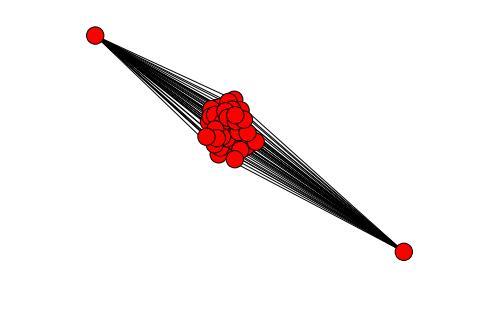In :
nx.draw_circular(g_nc1)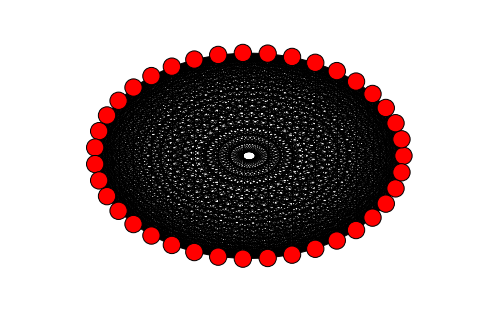In :
nx.draw_spectral(g_nc1)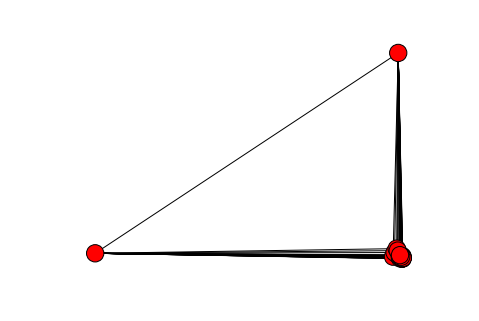In :
nx.draw_shell(g_nc1)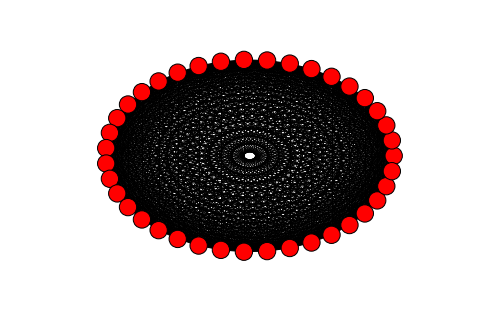In :
nx.draw_random(g_nc1)